# SLAM经典｜Fast-Lio2论文与代码详解

0收藏

### 1.系统框架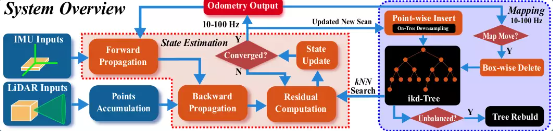### 2.状态方程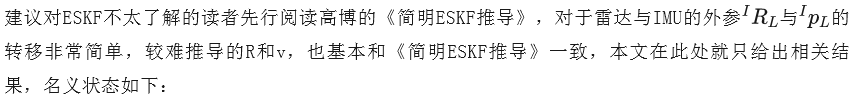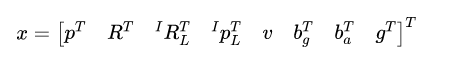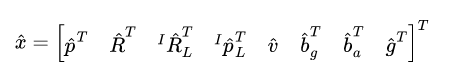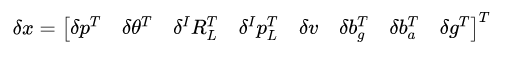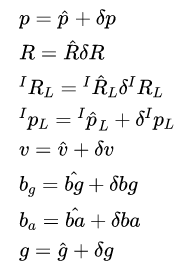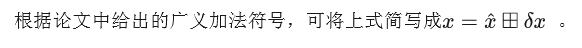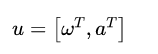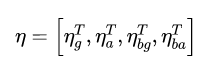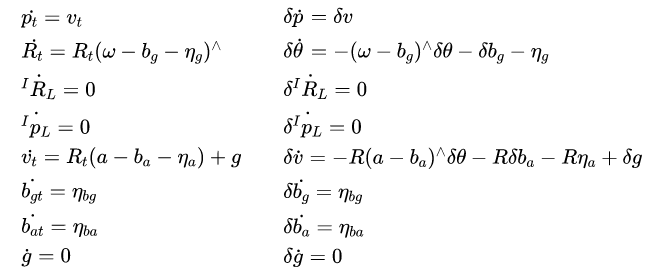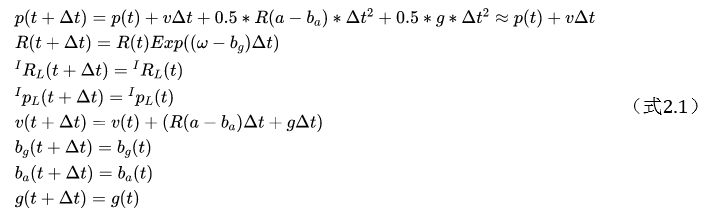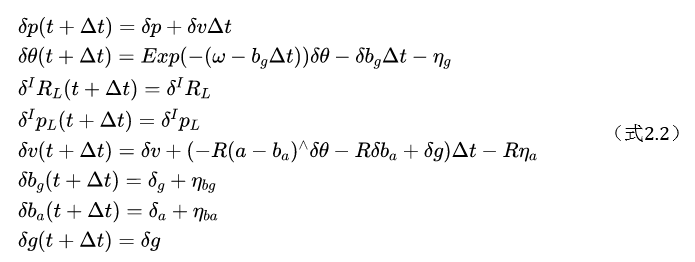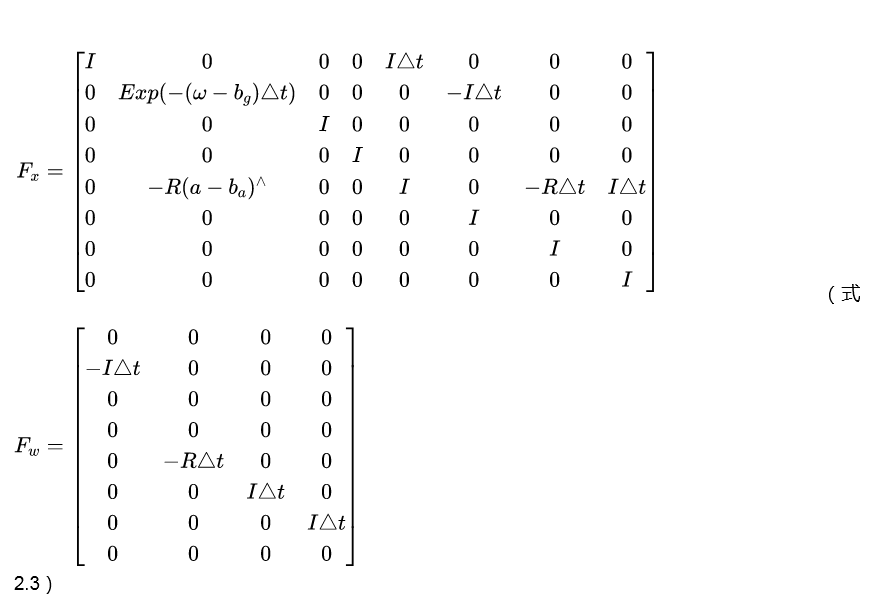### 3.向前传播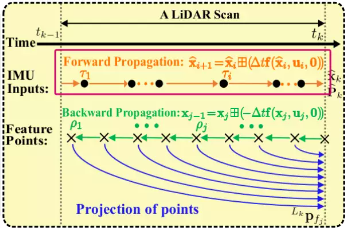`` UndistortPcl(meas, kf_state, *cur_pcl_un_);``

`` kf_state.predict(dt, Q, in);``

`````` flatted_state f_ = f(x_, i_in);
//f()即get_f()
Eigen::Matrix<double, 24, 1> get_f(state_ikfom &s, const input_ikfom &in)
{
Eigen::Matrix<double, 24, 1> res = Eigen::Matrix<double, 24, 1>::Zero();
vect3 omega;
in.gyro.boxminus(omega, s.bg); // 得到修正后的imu的角速度
vect3 a_inertial = s.rot * (in.acc-s.ba);
for(int i = 0; i < 3; i++ ){
res(i) = s.vel[i];      //对应p=p+vdt中的v
res(i + 3) =  omega[i]; //对应R=R*Exp(w-bg)dt中的w-bg
res(i + 12) = a_inertial[i] + s.grav[i]; //v=v+[R(a-ba)+g]dt中的R(a-ba)+g
}
return res;
}
x_.oplus(f_, dt);
//x_.oplus(f_, dt)完成了乘dt并广义自加``````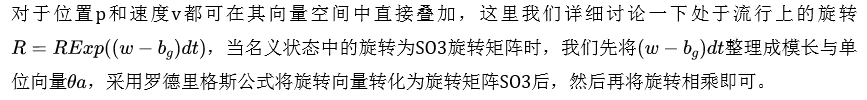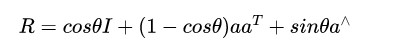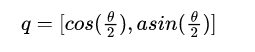`````` //代码有所简化，变量定义与上式不尽相同，但实际上cos_sinc_sqrt提供了两种计算方法，
//当1/2theta较大时，直接调用库函数求sin和cos即可
//因为这里都是小量，一般采用另外一种基于泰勒展开的数值计算方法，有兴趣的读者可以自己算一遍
std::pair<scalar, scalar> cos_sinc_sqrt(const scalar &x2){
using std::sqrt;
using std::cos;
using std::sin;
if(x2>=taylor_n_bound) {
// slow fall-back solution
scalar x = sqrt(x2);
return std::make_pair(cos(x), sin(x)/x); // x is greater than 0.
}
static scalar const inv[] = {1/3., 1/4., 1/5., 1/6., 1/7., 1/8., 1/9.};
scalar cosi = 1., sinc=1;
scalar term = -1/2. * x2;
for(int i=0; i<3; ++i) {
cosi += term;
term *= inv[2*i];
sinc += term;
term *= -inv[2*i+1] * x2;
}
return std::make_pair(cosi, sinc);
}``````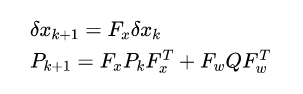``````// F_x1，f_w_final的计算与式2.3是一致的
P_ = (F_x1) * P_ * (F_x1).transpose() + (dt * f_w_final) * Q * (dt * f_w_final).transpose();``````

### 4.反向传播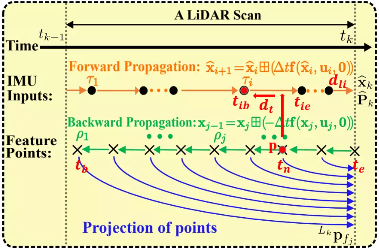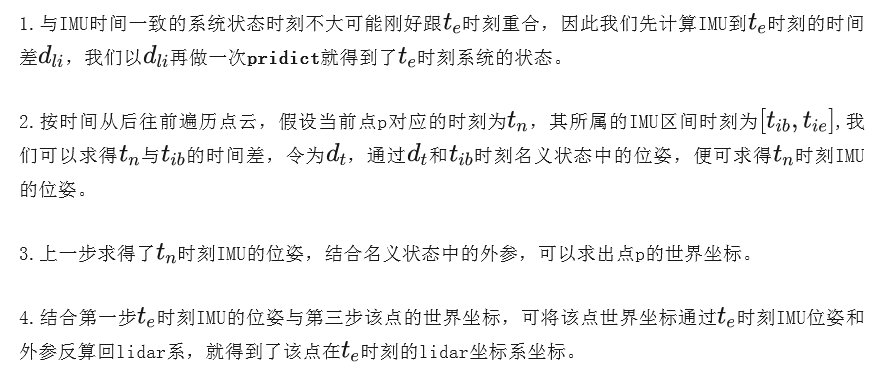`````` M3D R_i(R_imu * Exp(angvel_avr, dt));//点所在时刻imu的旋转
V3D P_i(it_pcl->x, it_pcl->y, it_pcl->z);//点所在时刻的位置(雷达坐标系下)
V3D T_ei(pos_imu + vel_imu * dt + 0.5 * acc_imu * dt * dt - imu_state.pos);
//P_compensate：补偿后点的坐标
//R_i，pos_imu，为点所在时刻imu的旋转与位置
V3D P_compensate = imu_state.offset_R_L_I.conjugate() *
(imu_state.rot.conjugate() * (R_i * (imu_state.offset_R_L_I * P_i + imu_state.offset_T_L_I) + T_ei)
- imu_state.offset_T_L_I);
//P_compensate为补偿后t_e时刻点的lidar系坐标，我们直接来看P_compensate的计算过程
//1：P_I=imu_state.offset_R_L_I * P_i + imu_state.offset_T_L_I->将t_n时刻雷达系下的点P_i 转换到imu系，得到点P_I
//2：P_w=R_i *P_I+pos_imu + vel_imu * dt + 0.5 * acc_imu * dt * dt->在t_n时刻下将点P_I转到世界得到点P_w
//3：P_ie=imu_state.rot.conjugate()*P_w-- imu_state.pos ->将将t_n时刻的点P_w转到最后时刻t_e下的imu坐标系得到点P_ie
//4：P_compensate=imu_state.offset_R_L_I.conjugate() *P_ie -imu_state.offset_T_L_I ->在t_e时刻下，将点P_ie转的雷达坐标系得到点P``````### 5.观测模型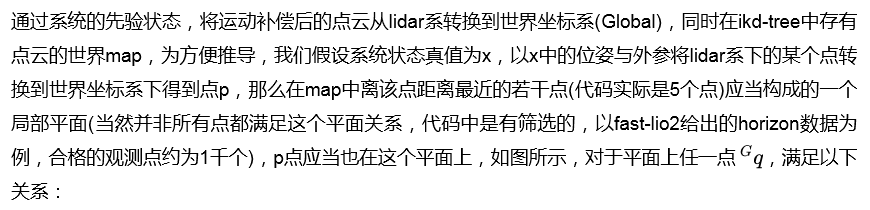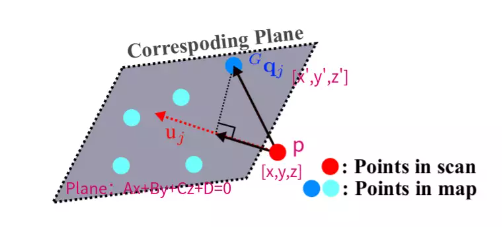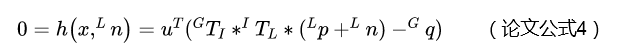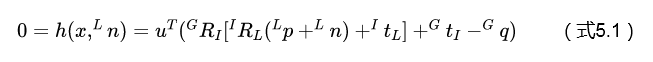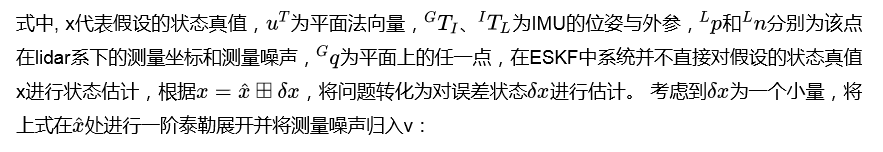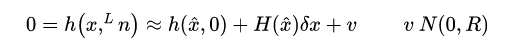（式5.2）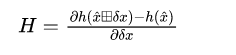（式5.3）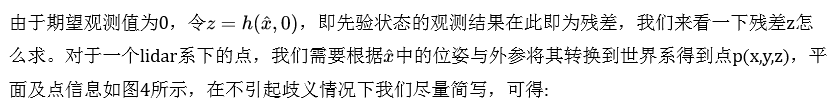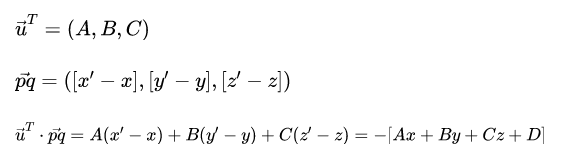``void h_share_model(state_ikfom &s, esekfom::dyn_share_datastruct<double> &ekfom_data)``

`````` //lidar坐标系下的点
V3D p_body(point_body.x, point_body.y, point_body.z);
//将点转换至世界坐标系下
V3D p_global(s.rot * (s.offset_R_L_I * p_body + s.offset_T_L_I) + s.pos)``````

`````` //point_world=p_global
ikdtree.Nearest_Search(point_world, NUM_MATCH_POINTS, points_near, po intSearchSqDis);``````

``esti_plane(pabcd, points_near, 0.1f)``

`````` //对比式5.4,注意这个式子pd2在最后是加了负号的
float pd2 = pabcd(0) * point_world.x + pabcd(1) * point_world.y + pabcd(2) * point_world.z + pabcd(3);``````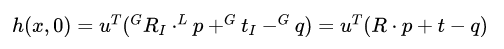（式5.5）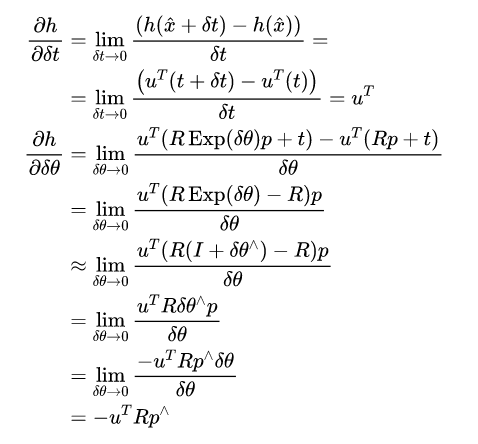（式5.6）由于代码里状态量中的旋转量均是四元数形式，注意到上式如果R是四元数是不方便直接计算的。当然，可以将四元数转化成旋转矩阵的形式再进行计算，也可以将5.6的结果进一步转化成代码的形式再代入四元数进行运算：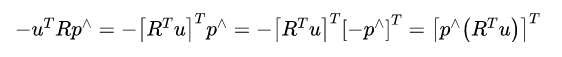（式5.7）

`````` //代码放在中间方便读者对照
//point_crossmat：点在imu坐标系下的坐标变成反对称^形式
//point_be_crossmat:点在lidar坐标系下点的反对称^形式
//norm_vec为法向量
//VEC_FROM_ARRAY----求向量的转置
V3D C(s.rot.conjugate() * norm_vec);
V3D A(point_crossmat * C);
V3D B(point_be_crossmat * s.offset_R_L_I.conjugate() * C);
//无外参下的H,VEC_FROM_ARRAY(A)-------->(式5.7)
ekfom_data.h_x.block<1, 12>(i, 0) << norm_p.x, norm_p.y, norm_p.z, VEC_FROM_ARRAY(A), 0.0, 0.0, 0.0, 0.0, 0.0, 0.0;
//有外参下的H
//VEC_FROM_ARRAY(A)-------->(式5.9)
//VEC_FROM_ARRAY(B)-------->(式5.10)
//VEC_FROM_ARRAY(C)-------->(式5.8)
ekfom_data.h_x.block<1, 12>(i, 0) << norm_p.x, norm_p.y, norm_p.z, VEC_FROM_ARRAY(A),VEC_FROM_ARRAY(B), VEC_FROM_ARRAY(C);``````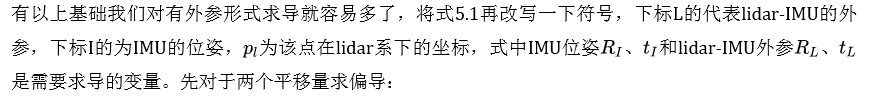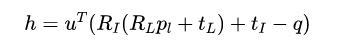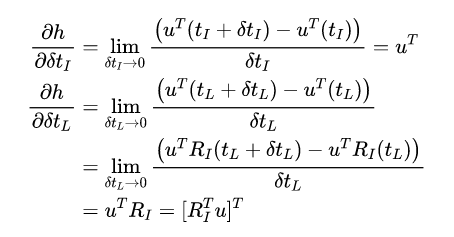（式5.8）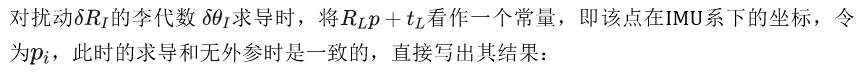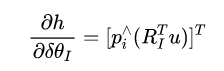（式5.9）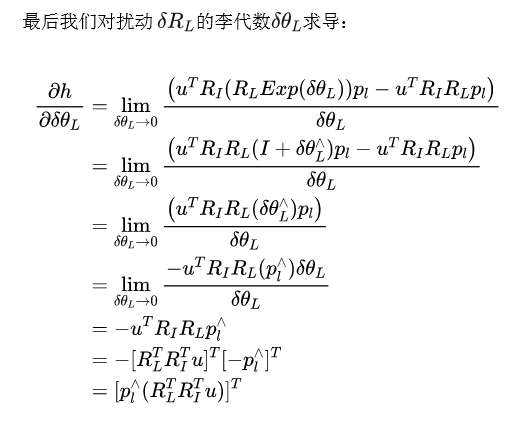（式5.10）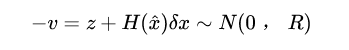（式5.11）

### 6.迭代更新

Fast-Lio2采用IEKF进行更新，由于观测模型中通过一阶泰勒展开进行了线性化近似(式5.2)，当线性化点与真值点距离较远时，线性化误差较大，当线性化点离真值越近时，线性化误差越小，缓解这一问题的方法就是在更新过程中，通过迭代进行重复观测，逐渐逼近最优线性化点，降低非线性带来的误差，提高估计精度。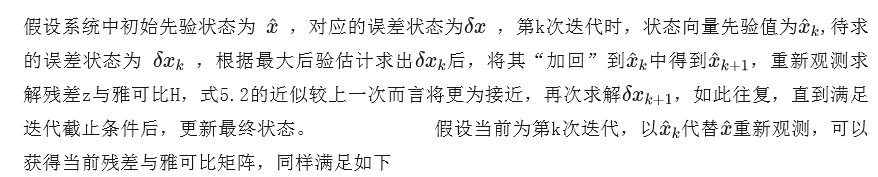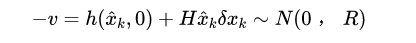（式6.1）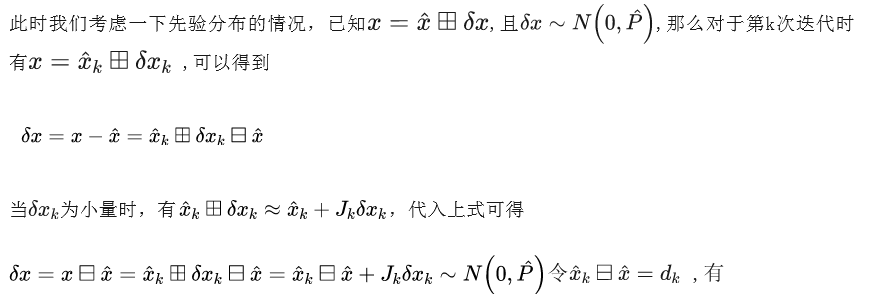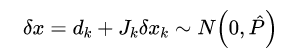（式6.2）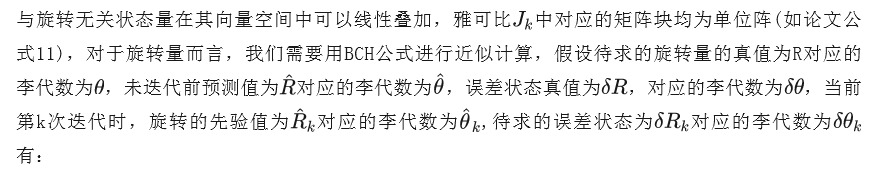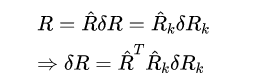（式6.3）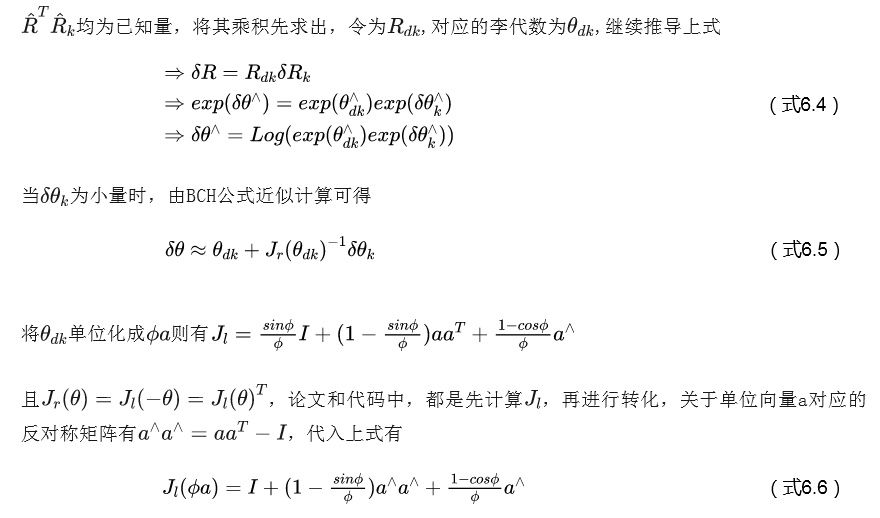计算过程代码如下

`````` //A_matrix()求J
Eigen::Matrix<typename Base::scalar, 3, 3> A_matrix(const Base & v){
Eigen::Matrix<typename Base::scalar, 3, 3> res;
double squaredNorm = v * v + v * v + v * v;
double norm = std::sqrt(squaredNorm);
if(norm < MTK::tolerance<typename Base::scalar>()){
res = Eigen::Matrix<typename Base::scalar, 3, 3>::Identity();
}
else{
//注意这里squaredNorm是模的平方，v是没有单位化的向量，和式6.6是一致的
res = Eigen::Matrix<typename Base::scalar, 3, 3>::Identity() +
(1 - std::cos(norm)) / squaredNorm * hat(v) +
(1 - std::sin(norm) / norm) / squaredNorm * hat(v) * hat(v);
}
return res;
}``````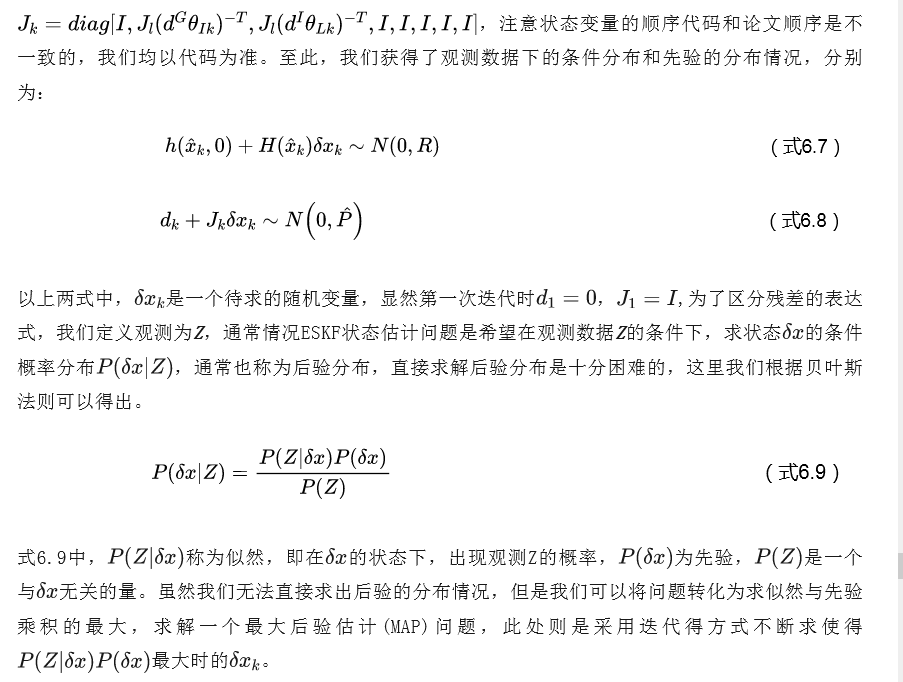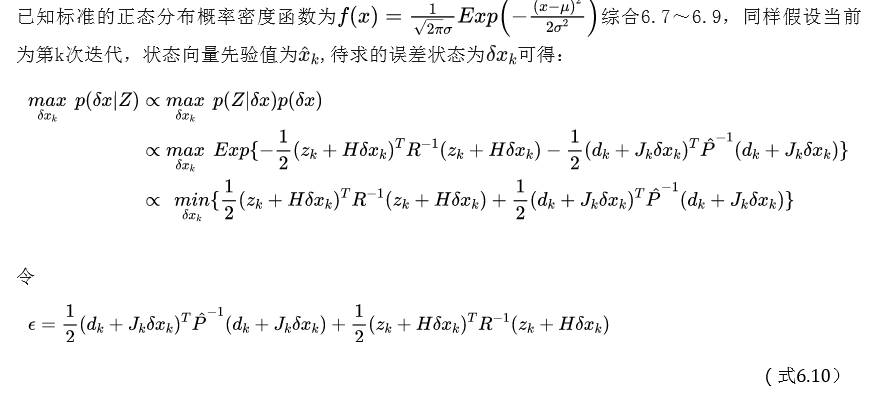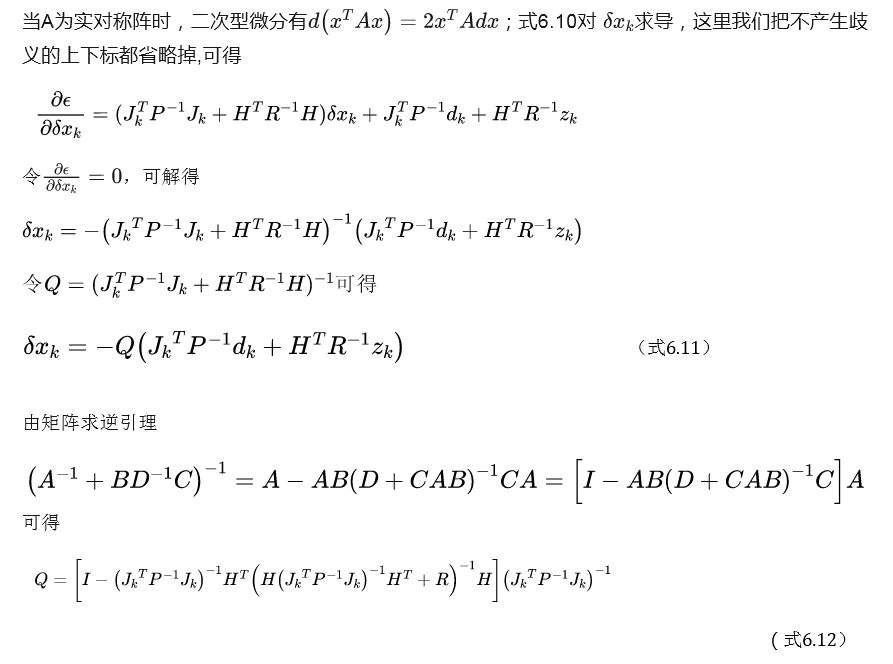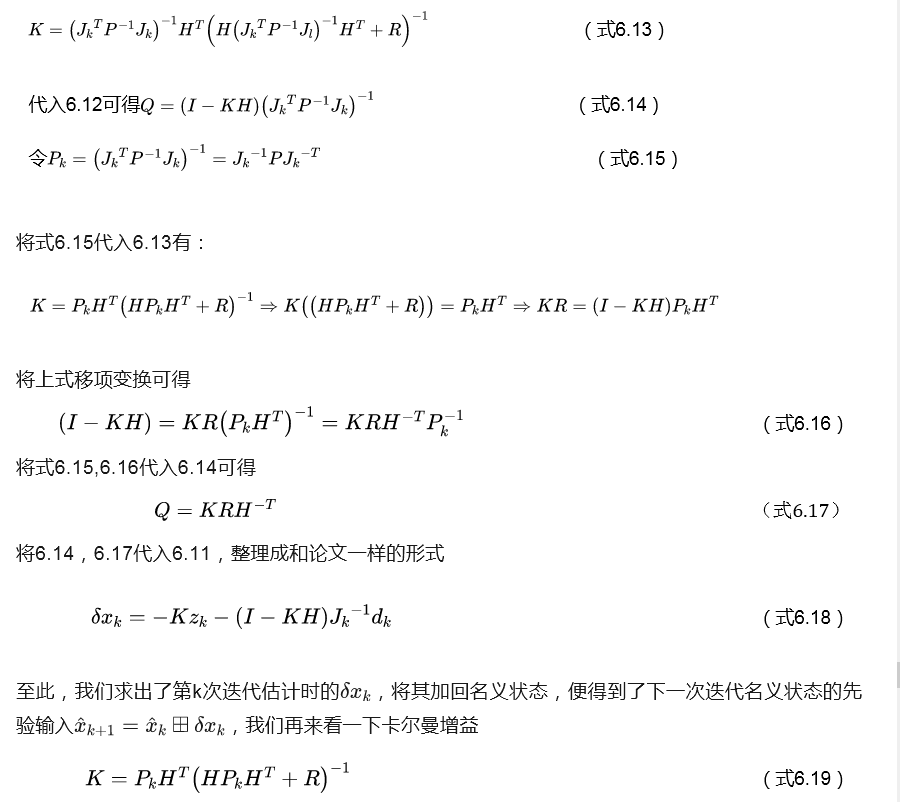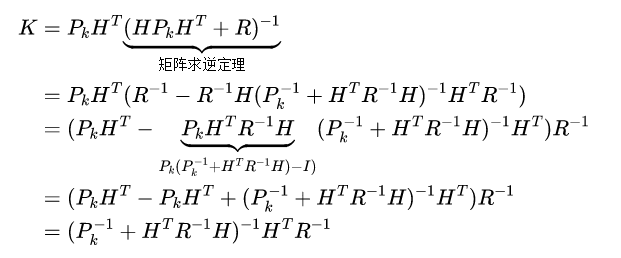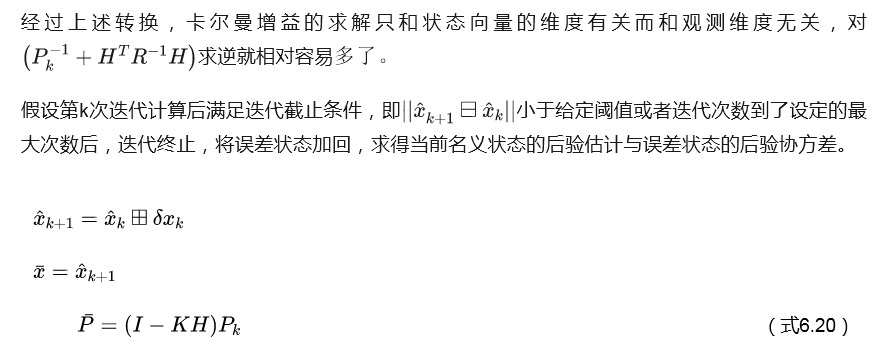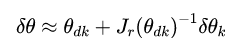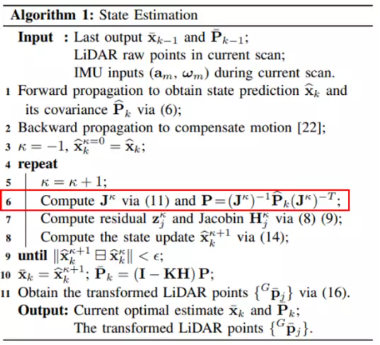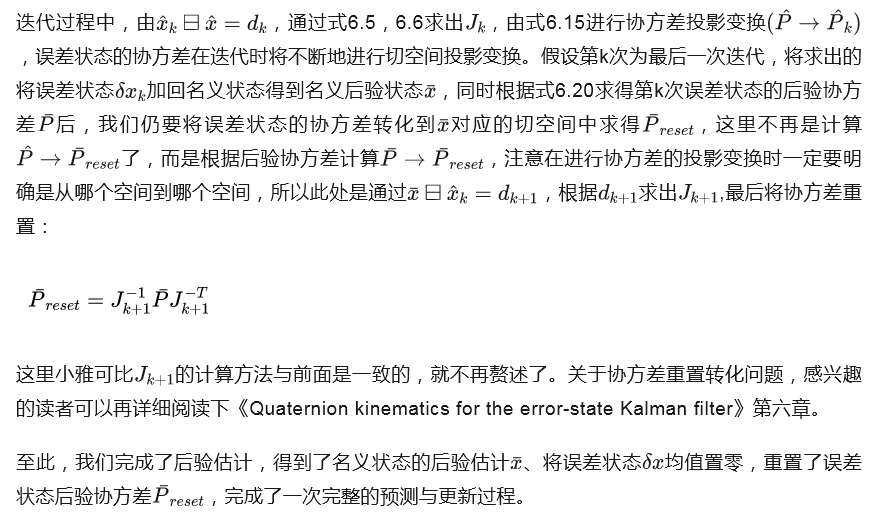### 7.主要参考

. Xu W, Zhang F. Fast-lio: A fast, robust lidar-inertial odometry package by tightly-coupled iterated kalman filter[J]. IEEE Robotics and Automation Letters, 2021, 6(2): 3317-3324.

. Xu W, Cai Y, He D, et al. Fast-lio2: Fast direct lidar-inertial odometry[J]. arXiv preprint arXiv:2107.06829, 2021.

 https://github.com/hku-mars/FAST_LIO

 半闲居士：简明ESKF推导

 鬼木士：FAST-LIO公式推导

 Quaternion kinematics for the error-state Kalman filter